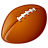# concatenation of fields

227
2
04-07-2020 10:05 PMNew Contributor

I am attempting to concatenate fields in a feature class.

The item to be calculated is T_GDE_con

and has 18 possible single entries, plus the concatenated entries, from 18 fields.

update([GDE_01C], [GDE_01D], [GDE_02C], [GDE_02D], [GDE_03C], [GDE_03D], [GDE_04D], [GDE_04E], [GDE_04F], [GDE_04G], [GDE_05C], [GDE_05D], [GDE_07C], [GDE_07D], [GDE_08C], [GDE_08D], [GDE_09C], [GDE_09D])

PRE LOGIC script code

def update(a, b, c, d, e, f, g, h, i, j, k, l, m, n, o, p, q, r):

strArray = [ ]

if a != ' ':

strArray.append(a + "/")

if b != ' ':

strArray.append(b + "/")

if c != ' ':

strArray.append(c + "/")

if d != ' ':

strArray.append(d + "/")

if e != ' ':

strArray.append(e + "/")

if f != ' ':

strArray.append(f + "/")

if g != ' ':

strArray.append(g + "/")

if h != ' ':

strArray.append(h + "/")

if i != ' ':

strArray.append(i + "/")

if j != ' ':

strArray.append(j + "/")

if k != ' ':

strArray.append(k + "/")

if l != ' ':

strArray.append(l + "/")

if m != ' ':

strArray.append(m + "/")

if n != ' ':

strArray.append(n + "/")

if o != ' ':

strArray.append(o + "/")

if p != ' ':

strArray.append(p + "/")

if q != ' ':

strArray.append(q + "/")

if r != ' ':

strArray.append(r + "/")

str = "".join(strArray)

str = str.strip()

str = str.lstrip("/")

str = str.rstrip("/")

return str

2 RepliesbyMVP Esteemed Contributor

Can we assume it didn't work? or do you have an error or a sample output?

You don't appear to be using the python parser for the field calculator

The script will probably fail if any of the inputs are not text/string and null values in the fields will cause issues as well.

Beyond that, the expression could be simplified if you can answer the questions on the first linebyMVP Esteemed Contributor

I don't see what you are trying to accomplish, but besides that you might want to replace all those if statements with elif.  Also, str as a variable is problematic;  using a function name spells trouble for me.

That should just about do it....